# 2. Data Resource

In fact I haven’t found the appropriate database from online data service, I have tried a lot of online database and download csv or cxt files. But they can not be imported or opened by either Galicia or Lattice Miner, so I generate the random data by Galicia: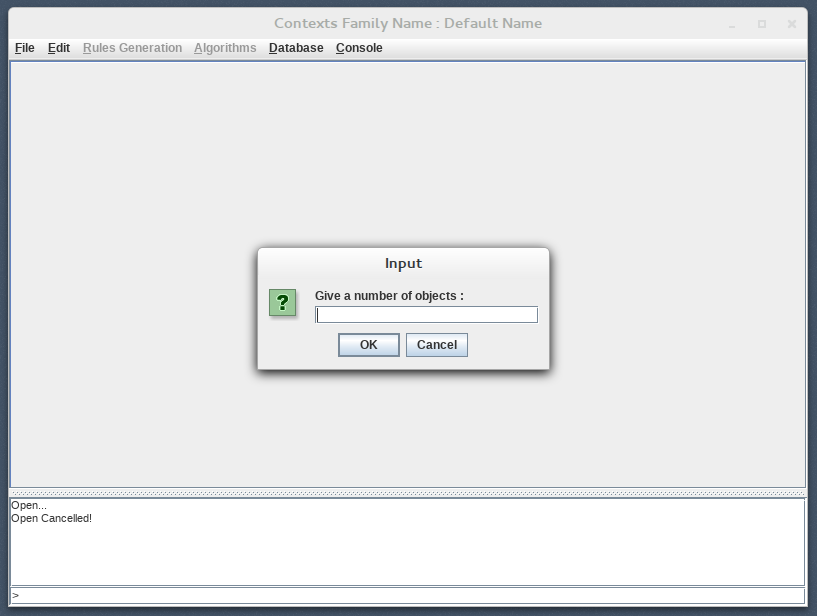Figure 1: Generate Random Database By Galicia

Input the number of objects and attributes, then a new binary table is generated: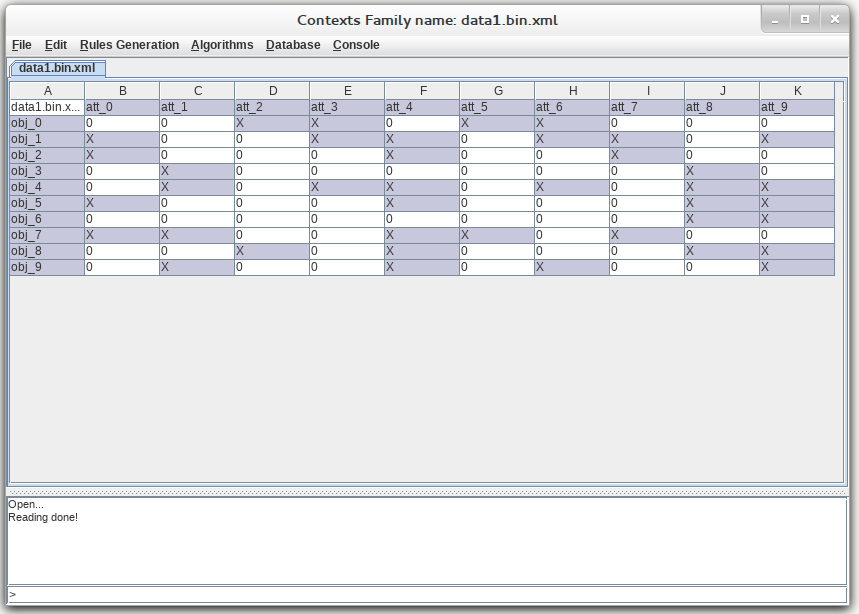Figure 2: Random Table in Galicia

# 3. Comparison

## 3.1 Galicia

### 3.1.1 Create or import data

Just as has mentioned above, we can create a new random table in galicia.

### 3.1.2 Construct the lattice

Algorithms->Construct the lattice: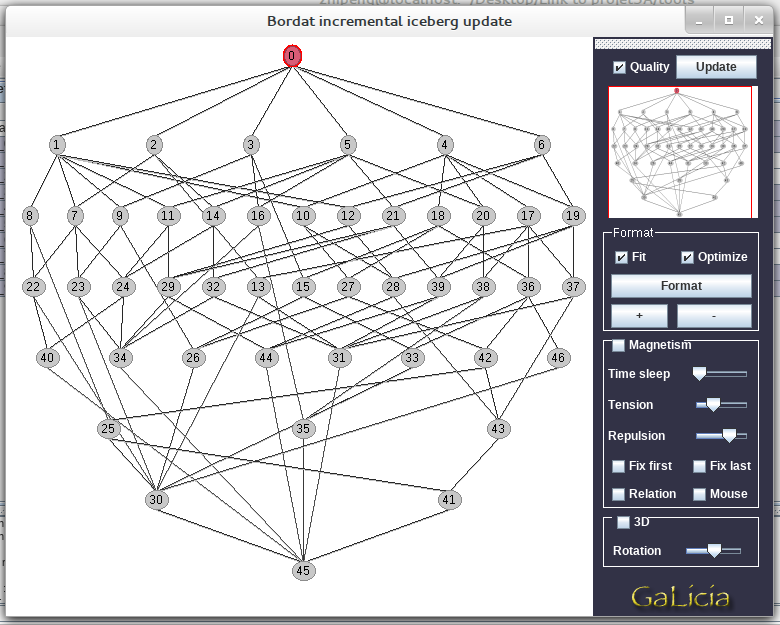Figure 3: Lattice Diagram Genereated by Galicia

There are some options to choose, for example, 3D display, which helps to better display the architecture.

### 3.1.3 Export Data

File->Export: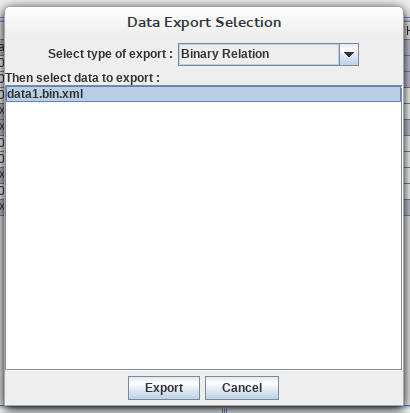Figure 4: Exporte Options Dialog

Remember to choose Binary Relation of the export type, because this type can be accepted by other software.

### 3.1.4 Calculation time

For example, if the number of Objects is 1000 and the number attributes is 20, if fill rate is 30%, the number of concepts(nodes) and calculation time is: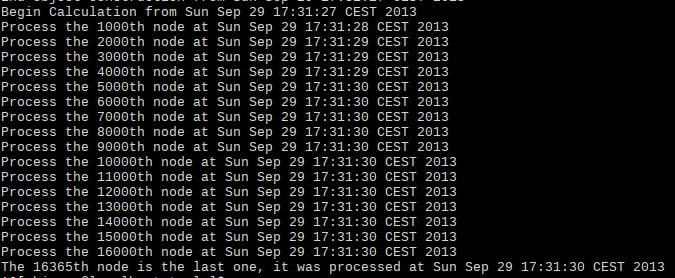Figure 5: Execution Log of Galicia (Running time)

So the entire calculation time is 3s. Here is the other calculation data:Table 1: Running Table of Galicia

The last example makes my computer restart.

I have noted the last line of command, which indicated that the number of concepts is more than 72k and time is more than 2m23s. Maybe it is because my pc is not powerful enough.

## 3.2 Lattice Miner

### 3.2.1 Create or import data

As we have generated the data by Galicia, we need to import the data file: data0.bin.xml.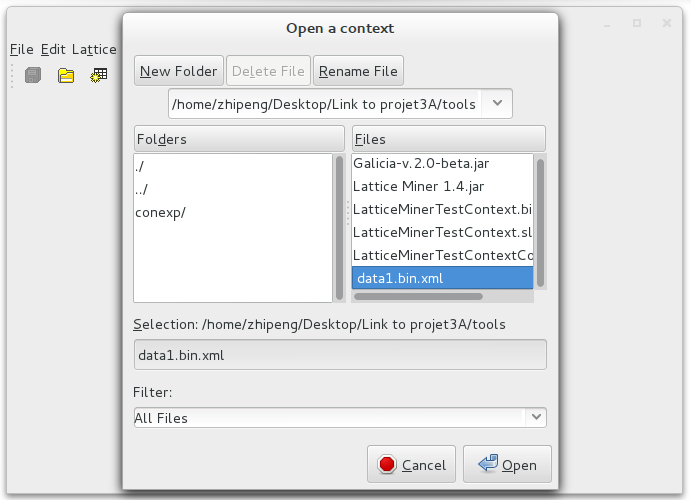Figure 6: Import Random Database in Lattice Miner

Open this file, the table will display.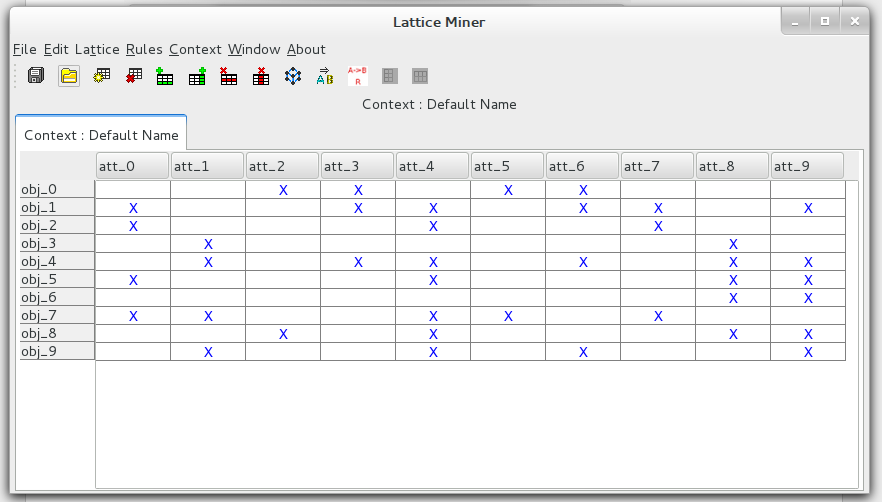Figure 7: Random Table in Lattice Miner

You can see it is exactly the same table as in Galicia (Please refer to 2. Data Resource)

### 3.2.2 Construct the lattice

Lattice -> Show Lattice: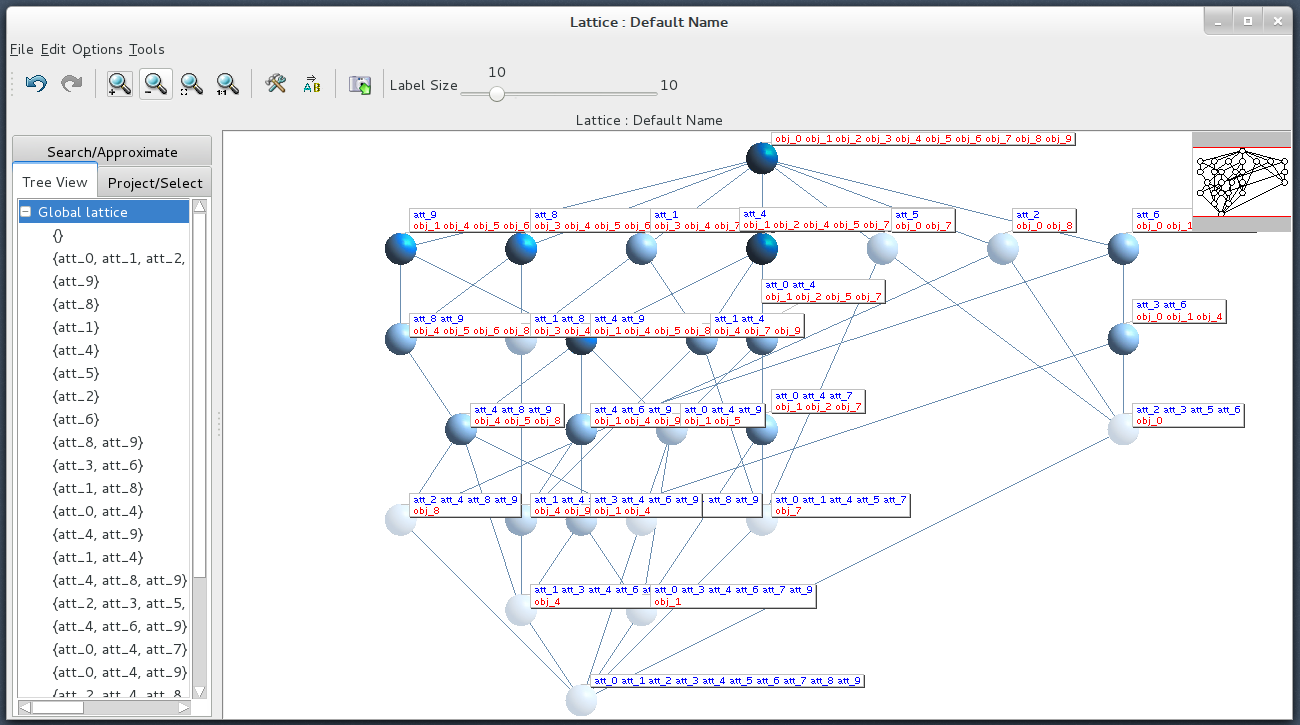Figure 8: Lattice Diagram in Lattice Miner

Apparently, there are many features for Lattice Miner:

• For each concept (each point in the picture), Lattice Miner labels its objects and attributes. For example, the second concept from right in the second line, its attribute is att_2, and it has two objects, obj_0 and obj_8. Of course, we can hide all labels, Edit -> Labels -> Hide all labels.
• Different colors for concepts. As we can see from the picture above, the colors of concepts vary according to the number of its objects. For example, the concept at top, it has nine objects, and it is darker than the concept at bottom, which has no objects.
• If a concept is clicked, the diagram will change. All its parent concept and child concept will be zoomed in and other concepts will fade. Like this: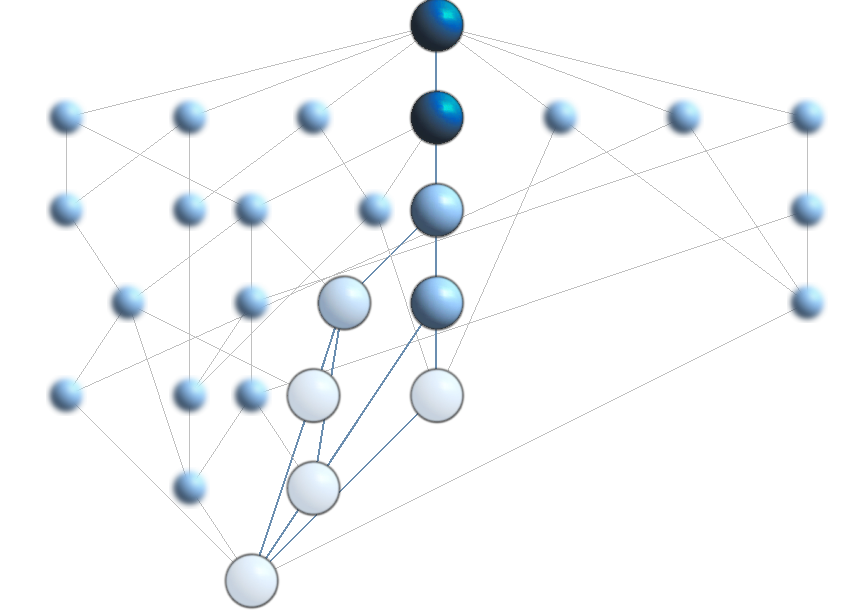Figure 9.1: Lattice Diagram1 with different concept chosen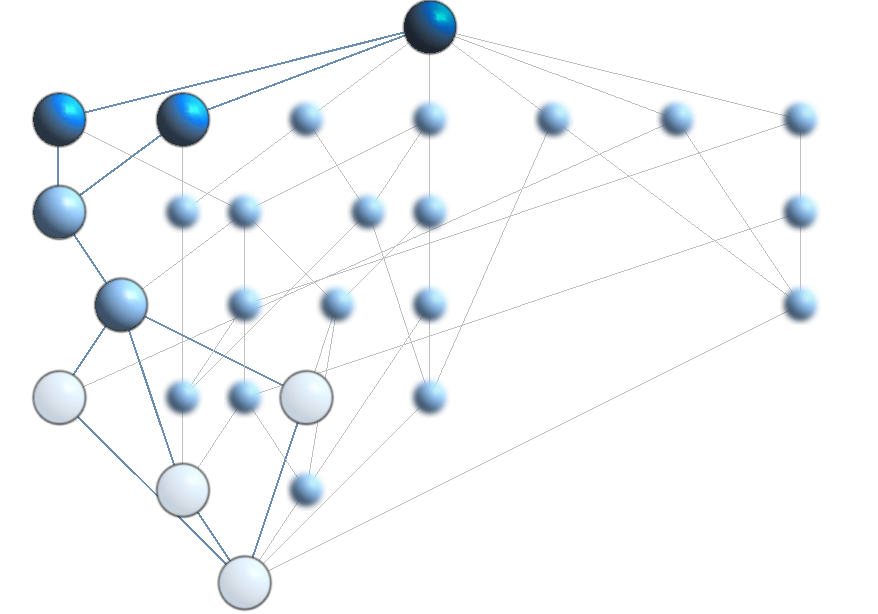Figure 9.2: Lattice Diagram2 with different concept chosen

### 3.2.3 Export data

File -> Save as.Figure 10: Export Options in Lattice Miner

There are four types for export, .slf, .bin.xml, .cex et .lmb.

### 3.2.4 Calculation time

I tried to use data0.bin.xml~data1.bin.xml, which were used as test data for Galicia. But unexpectedly, Lattice Miner can not calculate many concepts.

For example, when there are 1000 objects, 10 attributes and 20% as fill rate, Lattice Miner give out a warning like this: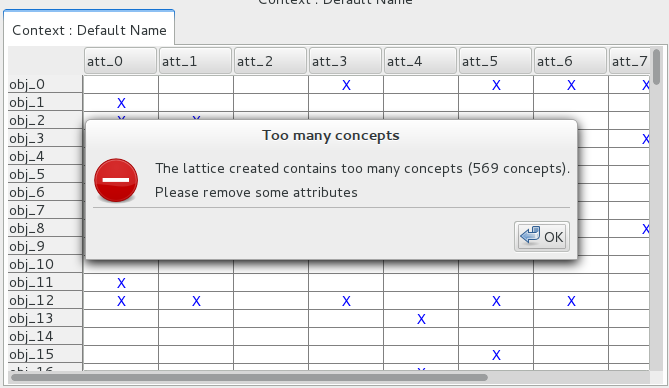Figure 11: Running warning in Lattice Miner

So here is the calculation time table:Table 2: Lattice Miner Running time table

The last two example makes Lattice Miner unresponded.

## 3.3 Open FCA

OpenFCA Project comprises a set of tools for performing FCA (Formal Concept Analysis) activities, such as context creation, lattice visualization and attribute exploration.

### 3.3.1 Create or import data

Different from other tools, OpenFCA is a web based platform, it use .con file to save database and don’t accept other formats of files. So in order to create big data, I analyze the basic structure of .con file, and then generate .con file by sript language, such as Python.

For example, a simple .con file and the core python code could be like this,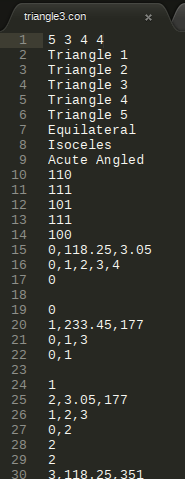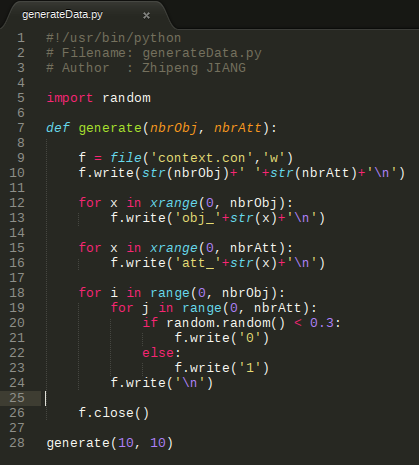Figure 12: Raw Data Structure and Python Code

In the python script, I have generated 10*10 table, and fill the table with ‘1’ by the chance of 70%.

### 3.3.2 Construct the lattice

After the data is imported, we can see a table like this,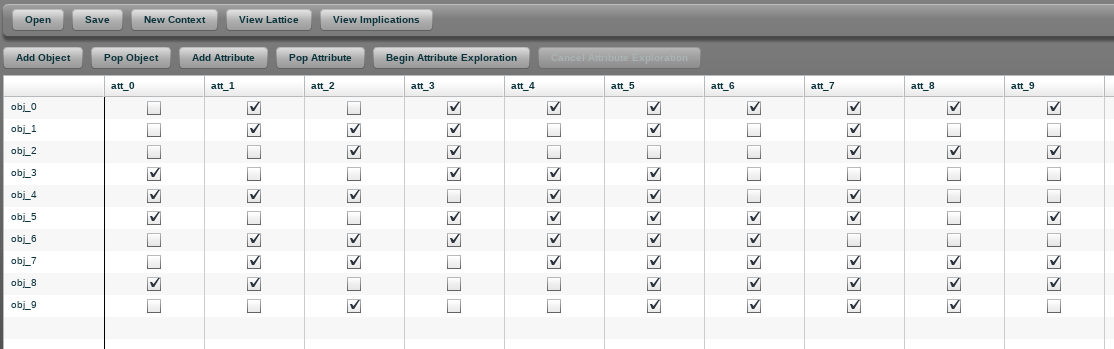Figure 13: Lattice Table in OpenFCA

Click the button View Lattice, the diagram will show up.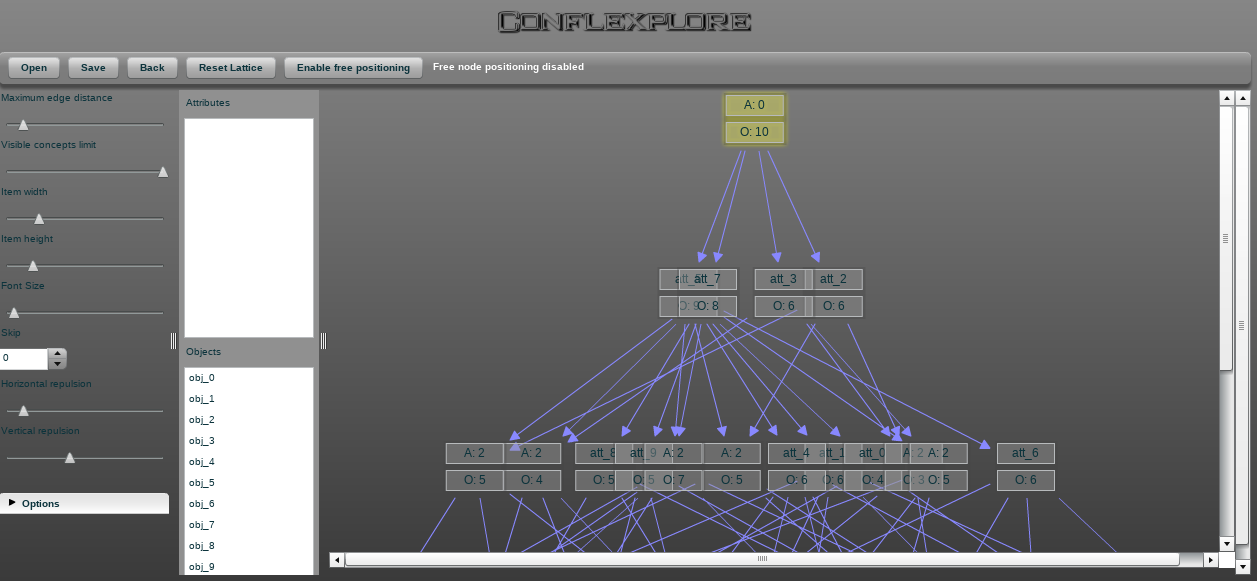Figure 14: Lattice Diagram in OpenFCA

Different from previous two Java program, this Flex tool is more beautiful and well designed. However, it doesn’t support many operations. Here are the main features.

When we move the mouse and hover it over each concept, all the objects and attributes will display in left. It is more useful and more clear than Galicia and Lattice Miner.

In the left side of page, there are some control bar, for example, Visible concepts limit, Item width, font size, etc.

At the top of this page, there are five buttons, open, save, reset, etc.

### 3.3.3 Export data

As OpenFCA only support .con file as database, there are no many choices for us.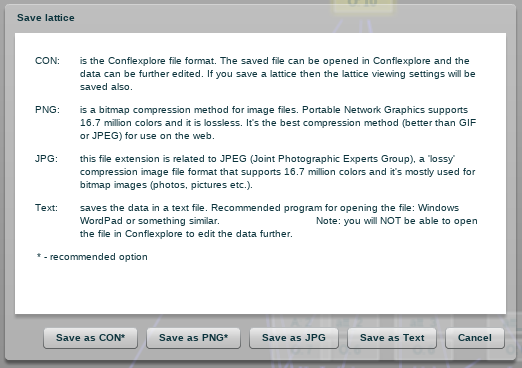Just as illustrated above, .con file is the best choice. .png and .jpg are just for presenting, they can not store data.

### 3.3.4 Calculation time

Unluckily, OpenFCA only supports 170 concepts at maximum. So we can not execute big data test. Generally, 101050% table takes one minutes. Perhaps, this limit is for speeding the respond time and improve user experience.

# 4. Conclusion

To sum up, in this report three tools are used and tested. Generally, we can conclude some key points as below:

• Functionality. By considering the support operations, file analyze and type support, calculation time, Galicia > Lattice Miner >> OpenFCA.

• Graphical user interface. There is no doubt that Flex+CSS is more powerful than Java UI opponents, OpenFCA > Lattice Miner > Galicia.